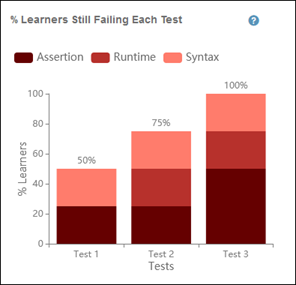## View Progress with Class Overview

Learner Analytics provide a way to assess learner progress using visual graphs. To access Learner Analytics, you must have a paid MATLAB® Grader™ license. See View Options under Get Started at MATLAB Grader for more information on features and license requirements.

To view these graphs, go to any problem and then click Class Overview.

### Status Summary

The status summary graph uses a pie chart to show the percentages of learners who have:

• Solved the problem.

• Not solved the problem but have made at least one submission.​

The percentages represent learners who are in the course.

The following image shows an example status summary graph. In it, you can see that 10 learners have accessed the problem. From that 10, 50 percent (5 learners) solved the problem, 40 percent (4 learners) did not, and no solutions were submitted by 10 percent (1 learner).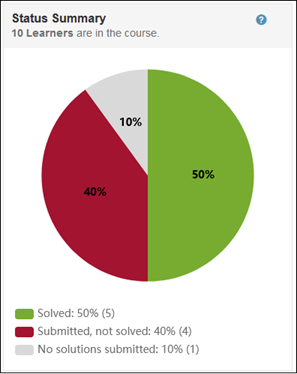### Submissions Required to Solve the Problem

The submissions required graph use a horizontal bar chart to show the number of submissions learners needed to solve the problem.

Key points:

• The graphs represent only learners who have solved the problem.

• The percentages represent the number of submissions it took per learner to submit a solution that solves the problem. The first solution that solves the problem is counted. For example, if a learner solves the problem in their third submission, then three submissions for that learner are counted, even if the learner continues to submit additional solutions, correct or not.

• If the assessment method is weighted, the problem is considered solved only when the learner’s score is 100%.

The following image shows an example submissions required graph. In it, you can see that the mean is 2, 20 percent of learners needed 4 submissions, 20 percent needed 3 submissions, and 60 percent needed 1 submission.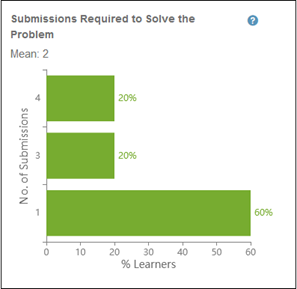### Average Submissions Required to Pass Each Test

The average submissions graph uses a vertical bar chart to show the average number of submissions required for learners to pass each test.​

Key points:

• The graph represents only learners who have solved the problem.

• The average for each test reflects when a learner permanently passes a test. For example, the learner passes a test for the first time on the second submission, fails it on the third and fourth submissions, and then passes it again on the fifth submission. If the learner continues to pass the test in subsequent submissions, MATLAB Grader records five as the number of times required to pass that test.

The following image shows an example of a average submissions graph. In it, you can see that test 1 required an average of 1.2 attempts to pass, test 2 required an average of 1.4 attempts to pass, and test 3 required an average of 2 attempts to pass.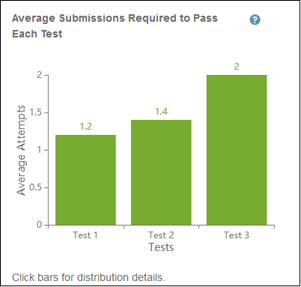For descriptions of each test and distribution details, click on the test bar.

### Submissions Made Attempting to Solve the Problem

The submissions made graph uses a horizontal bar chart to show the number of submissions learners have made attempting to solve the problem, by percentage of learners.

Key points:

• The percentages are of learners who have not yet solved the problem but have made at least one submission.​

• If the assessment method is weighted, the problem is considered solved only when the learner’s score is 100%.

The following image shows an example submissions made graph. In it, you can see that the top horizontal bar shows 25% of learners made 3 submissions each and the bottom bar shows 75% of learners made 2 submissions each.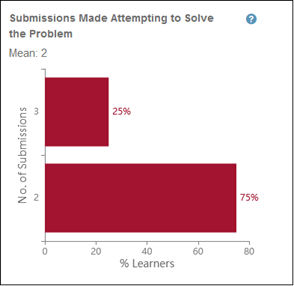### Learners Still Failing Each Test

The learners still failing graph uses a vertical bar chart to show the percentage of learners still failing each assessment test.

Key points:

• The percentages are of learners who have not yet solved the problem but have made at least one submission.​

• The graph reflects each learner’s most recent submission. For example, if a learner passed Test 2 in a previous submission but failed in their most recent submission, the graph counts the learner as failing the test.

• ​The graph distinguishes between the following types of errors:

• Assertion–The test condition failed. For example, if a test checks whether variable x equals the reference solution, but it does not, the test fails.

• Runtime–An error condition occurred when executing the code, requiring execution to stop. Possibilities include calling a MATLAB function with incorrect input parameters, negative indexing of a vector variable, or assigning an undefined function or variable. For script-based problems, runtime errors result in all tests failing.

• Syntax–The code contains a syntax error. For both script-based and function-based problems, syntax errors result in all tests failing.

• The following image shows an example of a learners still failing graph. In it, you can see bars for three tests, each of which learners are still failing with a various assortment of the possible errors.